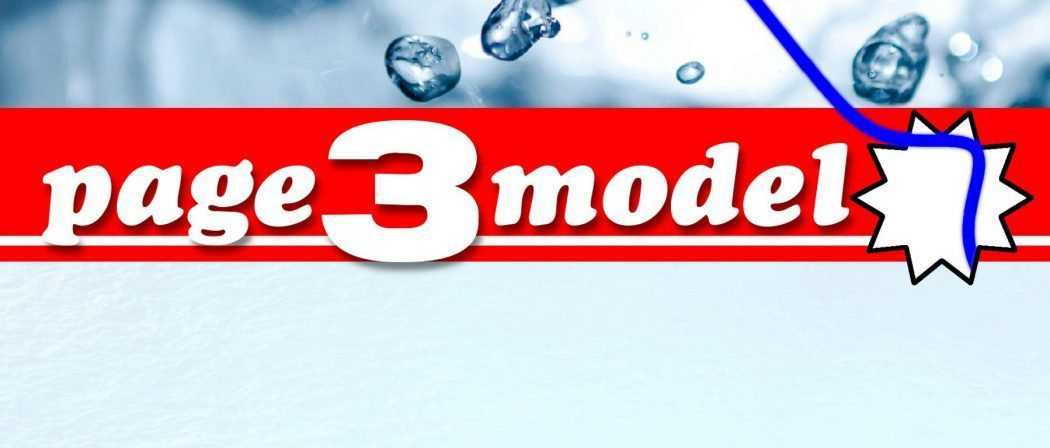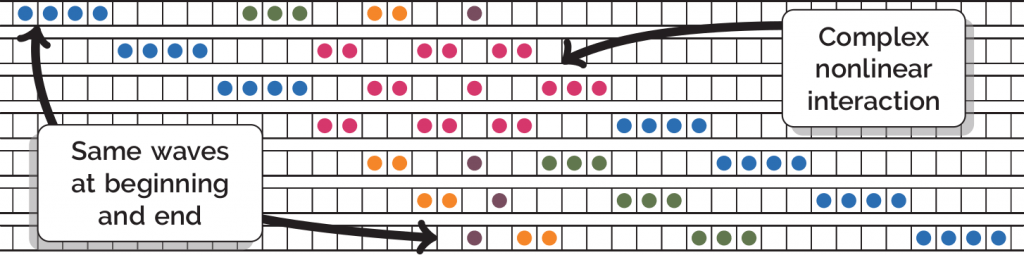# Page 3 model: Solitons

SolitonsSolitons are special analytic solutions to the nonlinear wave equations that turn up everywhere: from fluid dynamics to quantum mechanics to molecular biology. One such equation is the Korteweg–de Vries (KdV) equation for a 1D wave $u(x,t)$ evolving in time $t$: $\frac{\partial u}{\partial t}+6u\frac{\partial u}{\partial x}+\frac{\partial^3 u}{\partial x^3}=0.$ What makes solitons special is that they behave in many ways like solutions to the linear wave equation: propagating without losing their shape, and even interacting nicely:In 1990, Daisuke Takahashi and Junkichi Satsuma proposed a discrete model for solitons called the ball and box model. It consists of an infinite row of boxes, some of which contain a ball. A sequence of consecutive balls represents a wave. The pattern of waves after each time step is found by starting from the left and moving each ball to the next available empty box to its right.A row of $n$ consecutive balls behaves like a single wave, and moves to the right at a constant speed $n$. Several such waves, so long as they start sufficiently separated, will interact (in a possibly messy way), and then disperse, maintaining their original shapes overall.This model is fun to play with, but—surprisingly given its simplicity—it manages to capture a large amount of the interesting and unusual behaviour of the fluid waves modelled by the KdV equation.David is a final year PhD student at UCL studying geometric group theory. When not doing maths he can usually be found singing or playing the flute.
davidsheard.co.uk/    + More articles by David